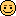## Recommended Posts

how do we find the square root of a recurring decimal eg) 1.3333*.

to what level of accuracy can we predict the square root, cube root, nth root etc?

##### Share on other sites

One method of computing square root is the following:

From: Wikipedia

The Taylor series of 1 + x about x = 0 converges for x ≤ 1 and is given byIt allows us to calculate the value to any degree of accuracy we wish. The method shown above is generalized as follows:

Taylor series

If N is an approximation to, a better approximation can be found by using the Taylor series of the square root function:Several calculation methods are shown in Wikipedia.

##### Share on other sites

how do we find the square root of a recurring decimal eg) 1.3333*.

to what level of accuracy can we predict the square root, cube root, nth root etc?

A repeating decimal will have a fractional form - the square root of that may be rational in which case I believe we could always find it exactly (might be a very long task) or it could be irrational in which case it can never be represented completely by a ratio of integers

Two obvious examples 1/3 and 1/9 --> 1/sqrt3 is irrational and our accuracy is what ever we want (ie just keep crunching the series with smaller and smaller increments) but never complete whereas 1/sqrt9 is 1/3 and there you have it - absolute accuracy.

Basically all the non-perfect squares are gonna have roots that are irrational, the same with cubes and higher powers. Doesn't matter if they are on the top or the bottom of the equation.

Sorry forgot - your example of 1.3333recurring is 4/3

square root (4/3) = 2/sqrt3

sqrt3 is irrational and so is your answer. You can never know it to full accuracy in any form of ratio representation

##### Share on other sites

You can never know it to full accuracy in any form of ratio representation

And yet apart from "full accuracy" you can achieve any degree of accuracy you want if you just bother to keep calculating long enough## Create an account

Register a new account